# Efficiency of Transformer

## Introduction of Efficiency of Transformer

Transformers form the most important link between supply systems and load. Transformer’s efficiency directly affects its performance and aging. The transformer’s efficiency, in general, is in the range of 95 – 99 %. For large power transformers with very low losses, the efficiency can be as high as 99.7%. The input and output measurements of a transformer are not done under loaded conditions as the wattmeter readings inevitably suffer errors of 1 – 2%. So for the purpose of efficiency calculations, OC and SC tests are used to calculate rated core and winding losses in the transformer. The core losses depend on the transformer rated voltage, and the copper losses depend on the currents through the transformer primary and secondary windings. Hence transformer efficiency is of prime importance to operate it under constant voltage and frequency conditions. The rise in the temperature of the transformer due to heat generated affects the life of transformer oil properties and decides the type of cooling method adopted. The temperature rise limits the rating of the equipment. The efficiency of transformer is simply given as: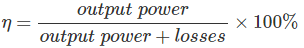• The output power is the product of the fraction of the rated loading (volt-ampere), and power factor of the load
• The losses are the sum of copper losses in the windings + the iron loss + dielectric loss + stray load loss.
• The iron losses include the hysteresis and eddy current losses in the transformer. These losses depend on the flux density inside the core. Mathematically,
Hysteresis Loss :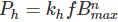Eddy Current Loss :Where kh and ke are constants, Bmax is the peak magnetic field density, f is the source frequency, and t is the thickness of the core. The power ‘n’ in the hysteresis loss is known as Steinmetz constant whose value can be nearly 2.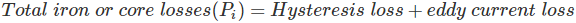• The dielectric losses take place inside the transformer oil. For low voltage transformers, it can be neglected.
• The leakage flux links to the metal frame, tank,etc. to produce eddy currents and are present all around the transformer hence called stray loss, and it depends on the load current and so named as ‘stray load loss.’ It can be represented by resistance in series to the leakage reactance.

## Efficiency Calculation of the Transformer

The equivalent circuit of transformer referred to primary side is shown below. Here Rc accounts for core losses. Using Short circuit(SC) test, we can find the equivalent resistance accounting for copper losses as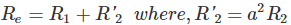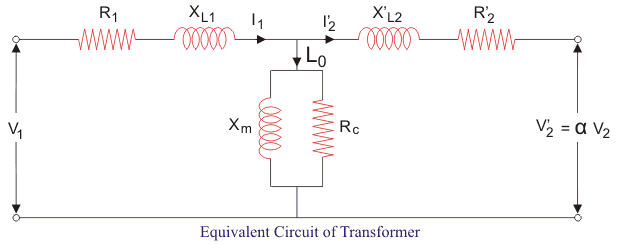Let us define x% be the percentage of full or rated load ‘S’ (VA) and let Pcufl(watts) be the full load copper loss and cosθ be the power factor of the load. Also, we defined Pi (watts) as core loss. As copper and iron losses are major losses in the transformer hence only these two types of losses are taken into account while calculating efficiency. Then the efficiency of transformer can be written as :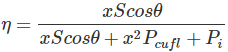The maximum efficiency (ηmax) occurs when the variable losses equal to the constant losses. Since the copper loss is load dependent, hence it is a variable loss quantity. And the core loss is taken to be the constant quantity. So the condition for maximum efficiency is :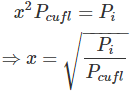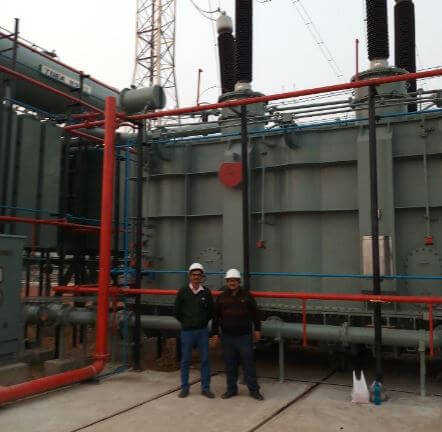Now we can write maximum efficiency as :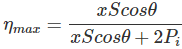This shows that we can obtain maximum efficiency at full load by proper selection of constant and variable losses. However, it is difficult to obtain maximum efficiency as copper losses are much higher than the fixed core losses.
The variation of efficiency with loading can be represented by figure below :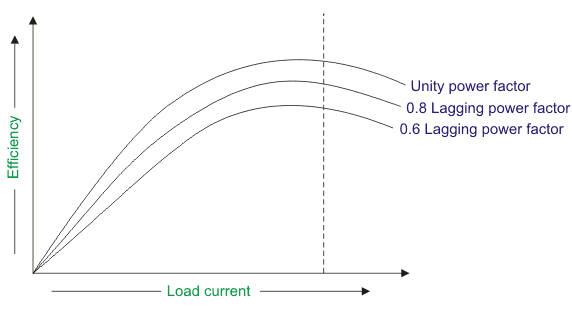We can see from the figure that the maximum efficiency occurs at unity power factor. And the maximum efficiency occurs at same loading irrespective of power factor of the load.

## All Day Efficiency of Transformer

It is an energy-based efficiency calculated for distribution transformers. Unlike power transformer which is switched in or out depending on the load handled by it, a distribution transformer loading continuously fluctuates for 24 hours a day. As core losses are independent of load, the all-day efficiency depends on the copper losses.We define it as the ratio of output energy delivered to input energy for a 24 hour cycle. High energy efficiencies are achieved by restricting core flux densities to lower values (as the core losses are dependent on flux density) by using relatively larger cross-section or larger iron/copper weight ratio.

Want To Learn Faster? 🎓
Get electrical articles delivered to your inbox every week.
No credit card required—it’s 100% free.